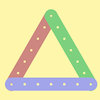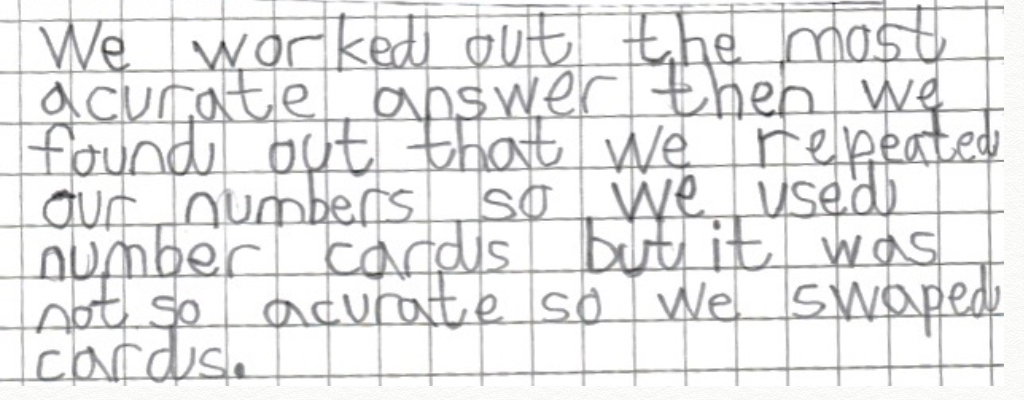#### You may also like### I'm Eight

Find a great variety of ways of asking questions which make 8.### Let's Investigate Triangles

Vincent and Tara are making triangles with the class construction set. They have a pile of strips of different lengths. How many different triangles can they make?### Noah

Noah saw 12 legs walk by into the Ark. How many creatures did he see?

# Two-digit Targets

##### Age 5 to 7Challenge Level
Many of you explained that you had concentrated on getting one of the five numbers at their 'best' to begin with because it was impossible to have all five at their best simultaneously.  We have summarised these solutions in the tables near the end of this page, but here they are in detail:

Roberto from Toucan School in Panama wrote:

First I did the closest number to 50 because it has only two options 49 or 51. Then I did the highest multiple of 5, which was 25 because you can only use numbers that end in 0 or 5. Then I did the smallest odd number, which is 01. Later, I did the highest even number, which is 86, and finally used the last two digits to make the highest odd number, which came out to be
73.

Nico, from the same school as Roberto, said:

First, I started with the closest number to 50, and it was either 51 or 49. Then I wrote the smallest odd number, which had to be 01. Later, I chose 35 for the largest multiple of 5. Finally, I used the last four digits I had to write 86 for the largest even number, and 27 for the largest odd number.

Bryan from Maadi British International School in Egypt sent in a picture of written work:Using number cards for this task can be very helpful - good thinking, Bryan!

Brooke from St Mary's CE Primary School in Slough, UK told us:

If you want to find any solutions that would fit, you need to look at what you are dealing with first. For example, you need to consider the largest even number. From 0-9, how would you tackle that? It's simple. You can use any two numbers, so go with the easiest two. 98. So you can't have 9 and you can't have 8 any more. Next, you need to consider the largest odd number. 75. Then, you need to consider the smallest odd number, which would be 13. After that, you need to look at the largest multiple of 5, which would be 60. You see, if I hadn't have looked at the options I would've come across that one and realised that I hadn't got a 5 or a 0. Finally, you need to look at the last one, which is the number closest to 50. You've only got two numbers left, so it would be 42.

Georgia from Woodlea Junior School in the UK wrote:

When I was doing this, I knew that I had to start with the largest multiple of 5 because multiples of 5 can only end in a 5 or a 0. Then, I did the smallest odd number because for that I knew that I should probably use my smallest digits because I needed the larger numbers for some of the other questions. After that, I did the number closest to 50 because I still had the 4 left, so I put that in the tens place because 40 is close to 50, and I put a number in the ones place as well. Finally, I did the biggest odd number and the biggest even number since they were the last two left, so I put my two smallest odd and even number in the ones place because I knew that I needed the bigger numbers for the tens place, and then I put the two other numbers in the tens place.

Amelia from Slip End village school, UK said:

I found that 94 was the largest even number and 87 the largest odd number. 23 was the smallest odd number and 60 was the biggest multiple of 5 and 51 was closest to 50.

You can see all these results, along with Brody's (who is from Coteford Junior School) and Ryder's (who is from Anderson's Creek Primary in Australia) in the tables below:

 Brooke Ryder LARGEST EVEN 98 98 largest odd 75 67 smallest odd 13 01 largest multiple of 5 60 35 nearest to 50 42 42

 Brady largest even 86 LARGEST ODD 97 smallest odd 01 largest multiple of 5 45 nearest to 50 32

 Roberto Nico Georgia Brady Ryder largest even 86 86 82 86 98 largest odd 73 27 73 97 67 SMALLEST ODD 01 01 01 01 01 largest multiple of 5 25 35 65 45 35 nearest to 50 49 49 49 32 42

 Bryan largest even 96 largest odd 75 smallest odd 13 largest multiple of 5 80 nearest to 50 42

 Roberto Nico Georgia Amelia largest even 86 86 82 94 largest odd 73 27 73 87 smallest odd 01 01 01 23 largest multiple of 5 25 35 65 60 nearest to 50 49 49 49 51

It is interesting that some of you decided that zero could only be a ones digit whereas others thought it could go in the tens column too.  I wonder whether you could convince us one way or the other.  Max from BRES gave the following which only uses zero in the ones column:

largest even number: 98
largest odd number: 75
smallest odd number: 13
largest multiple of 5: 60
number closest to 50: 42

So, we have many possible solutions to this challenge.  How do we judge which solution is 'best'?

Well, we could decide that 'best' means as near as possible to the ideal number which has each property. So, the ideals would be 98, 97, 13, 95 and 50 if we decide that zero can only be in the ones column. One way to judge how close a solution is to the ideal might be to work out the difference between the ideal number and the one you have. So, for example, for Max's solution: 98, 75, 13, 60, 42...

98 is ideal, therefore, the difference is zero.
75 is twenty-two away from the ideal 97.
13 is the ideal.
60 is thirty-five away from the ideal.
42 is eight away.
So, we could say that in total, the difference is sixty-five.

Is there a solution that is closer if we use this way of judging how good solutions are?
If you have come up with a different way of comparing solutions, please let us know.